# Volume of Pyramid

Volume of Pyramid
Go back to  'Volume'

 1 Introduction to Pyramids 2 Types of Pyramids 3 Definition of Volume of a Pyramid 4 Volume of a Pyramid calculator 5 Thinking out of the Box! 6 Solved Examples 7 Practice Questions 8 Important Notes 9 Maths Olympiad Sample Papers 10 Frequently Asked Questions (FAQs)

We at Cuemath believe that Math is a life skill. Our Math Experts focus on the “Why” behind the “What.” Students can explore from a huge range of interactive worksheets, visuals, simulations, practice tests, and more to understand a concept in depth.

Book a FREE trial class today! and experience Cuemath’s LIVE Online Class with your child.

## Introduction to Pyramids

Have you visited or seen pictures of the Great Pyramid of Giza

What shape is it?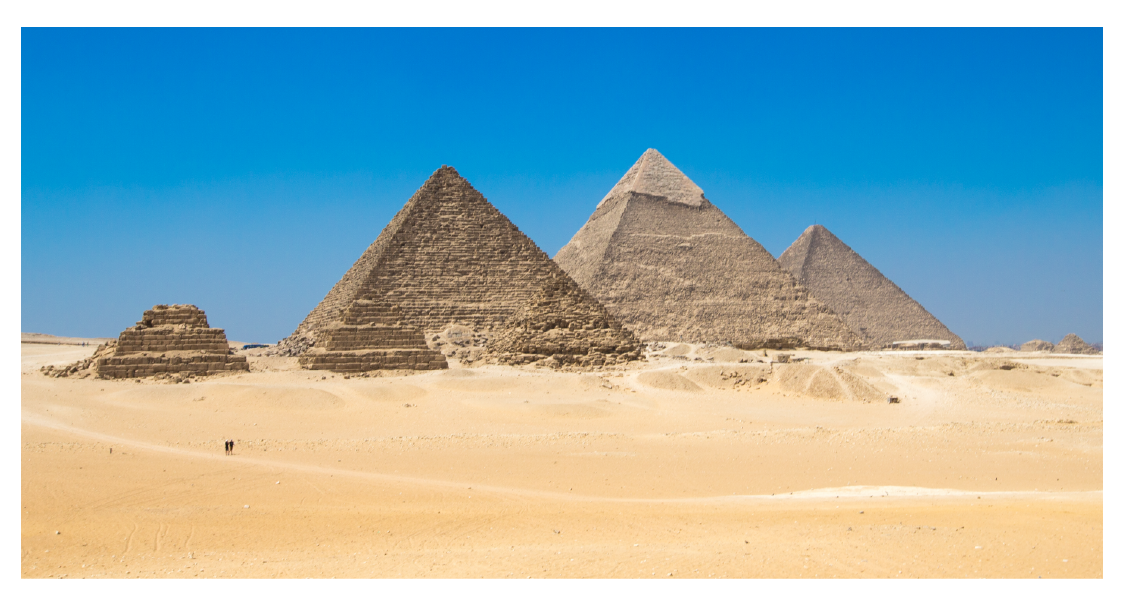Pyramids are solid shapes.

They have a polygon as their base and triangular faces that meet at the apex.

Apex is a vertex which is opposite to the base of the pyramid.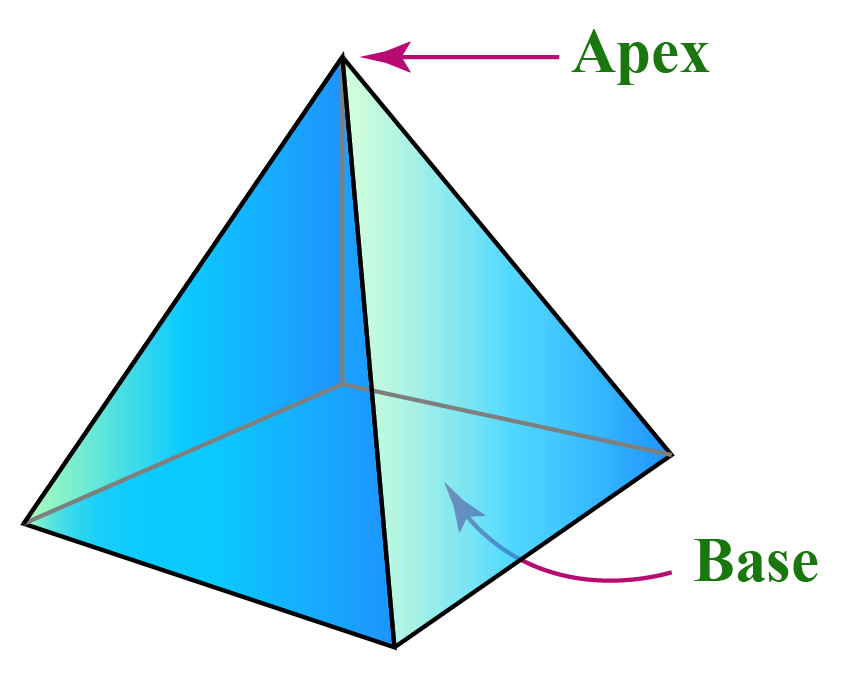## Types of Pyramids

We just learnt that a pyramid has a polygon as its base.

Pyramids can be classified into different types depending on their bases. They include:

1. Triangular Pyramid
3. Pentagonal Pyramid

Let's discuss explore them further.

### Triangular Pyramid

When the base of a pyramid is a triangle, we call it a triangular pyramid.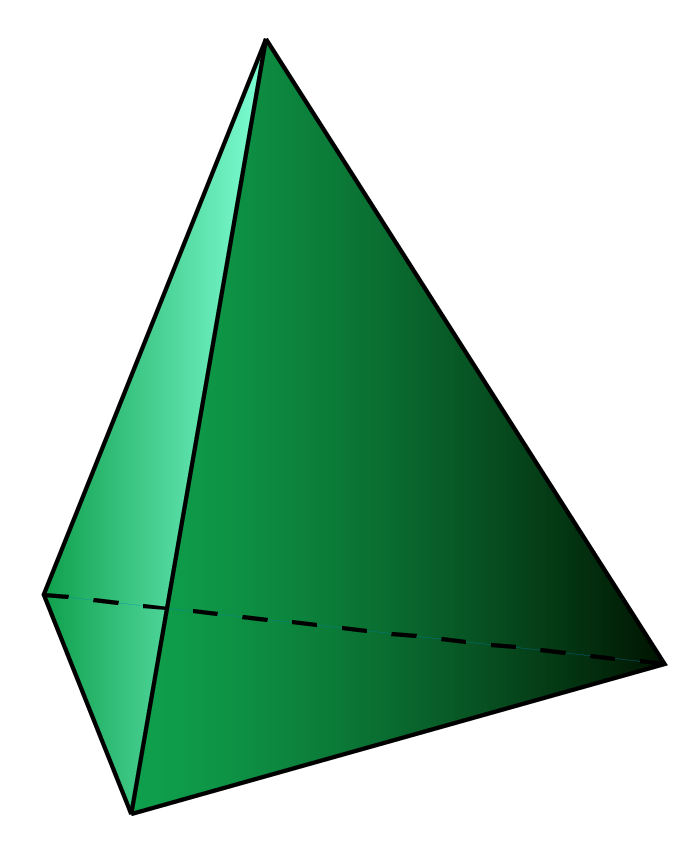Note: Tetrahedron is a triangular pyramid which has all its faces as triangles.

A Quadrilateral pyramid has quadrilaterals like a square or a rectangle as its base.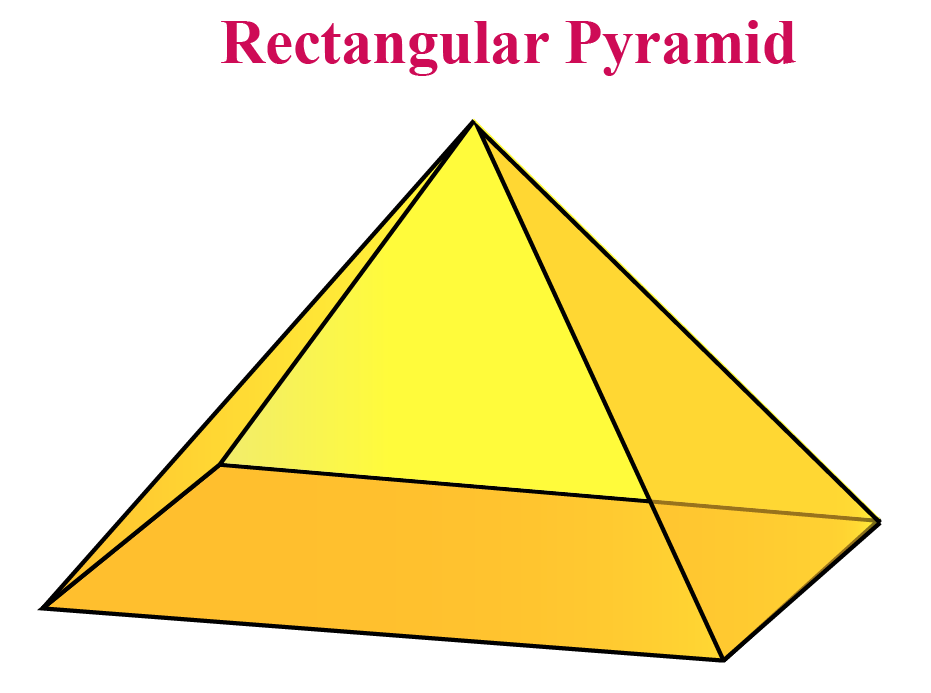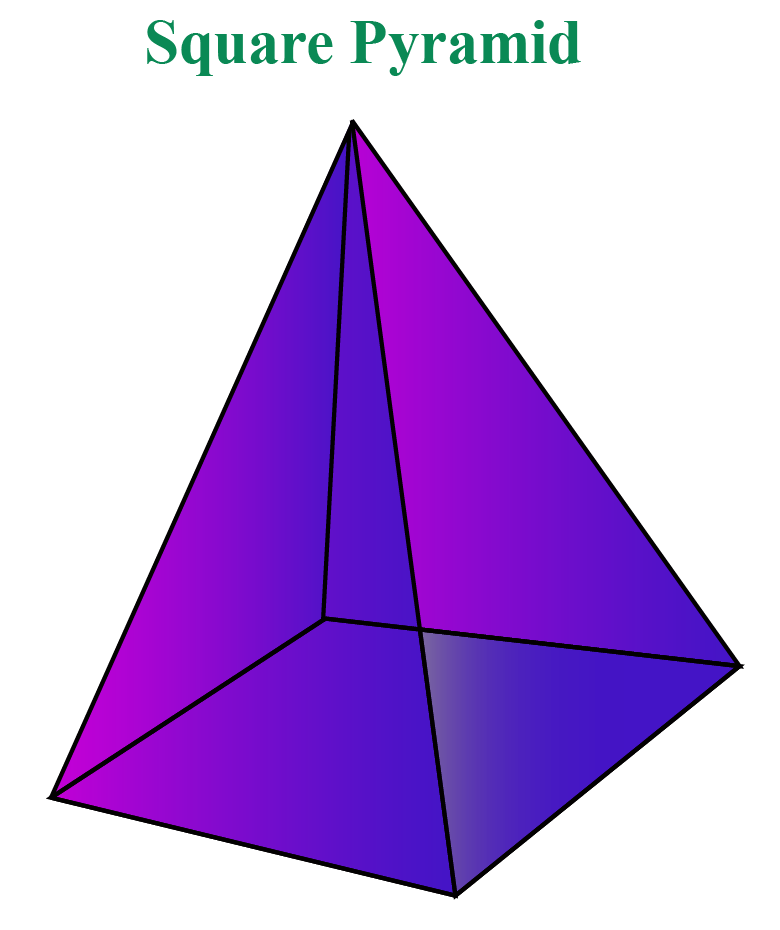### Pentagonal Pyramid

A pentagonal pyramid has a pentagon as its base.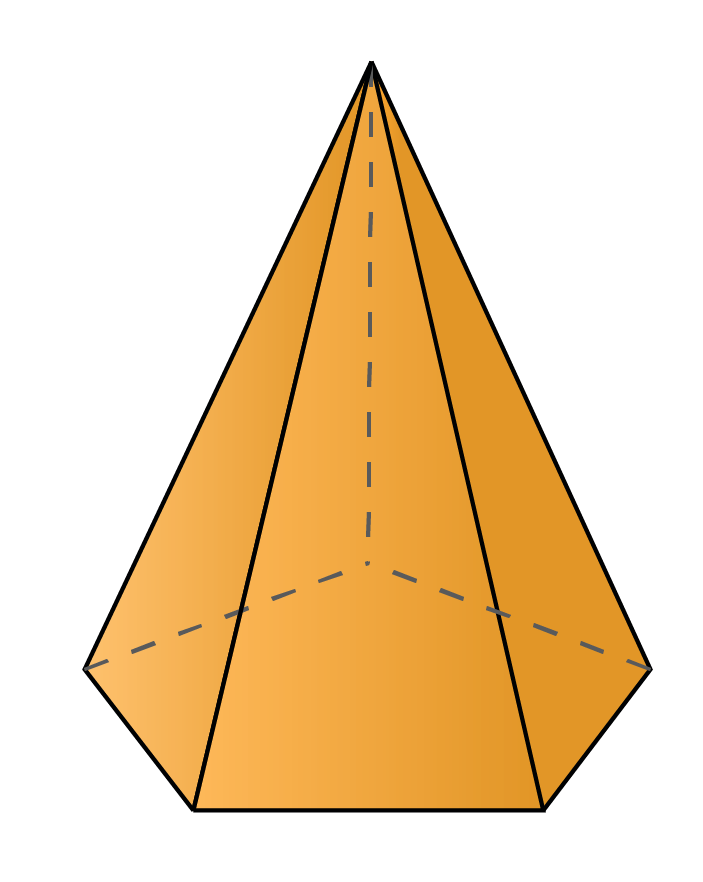If we know the area of a pentagon, we can calculate the volume of a pentagonal pyramid.

Here's a task for you. Can you draw a hexagonal pyramid?

## Definition of Volume of a Pyramid

Volume of a pyramid refers to the space enclosed between its faces.

Look at the simulation below where the nets of different pyramids fold to form a solid shape.

The amount of space enclosed by these faces is the volume of the pyramid.

The volume of a pyramid is one-third of the product of the area of the base and the height of the pyramid.

 \begin{align}\text{Volume} = \frac{1}{3} \times \text{Base Area} \times \text{Height}\end{align}

Consider a square pyramid.

We know that the base of this pyramid is a square.

Let the side of the square be $$a$$ and height of the pyramid be $$h$$.

Thus, the volume of the square pyramid is:

 \begin{align}\text{Volume} = \frac{1}{3} \times a^2 \times h \end{align}

We can use the same method to find the volume of a pyramid with any polygon as its base.

We know that the area of a polygon is given by the formula:

 \begin{align}\frac{1}{2} \times \text{Perimeter} \times \text{apothem} \end{align}

Note that apothem is the line segment from the center of a regular polygon to the midpoint of a side.

Once we find the base area of the polygon, we can apply the volume of a pyramid formula to calculate it.

Can you deduce a formula to calculate the volume of a pentagonal pyramid and a hexagonal pyramid?

Note that area of a pentagon is

\begin{align}\frac{1}{2} \times 5 \times (\text{edge length}) \times \text{apothem }\end{align}

($$\because\! \text{Perimeter of pentagon} \!=\!5\!\!\times\!\!\text{edge length}$$)

Therefore, Volume of a Pentagonal pyramid is

 \begin{align}\text{Volume} = \frac{5}{6} \times a \times s \times h \end{align}

Area of a hexagon is

\begin{align}\frac{1}{2} \times 6 \times (\text{edge length }) \times \text{apothem }\end{align}

($$\because \text{Perimeter of hexagon} \!=\!6 \!\times\! \text{edge length}$$)

Therefore, Volume of a Hexagonal pyramid is

 \begin{align}\text{Volume} = a \times s \times h \end{align}

Where;

• $$a$$ = apothem length
• $$s$$ = edge length of the polygon and
• $$h$$ = height of the pyramid

If apothem length is not known,

We can also use the area of a triangle formula to calculate the area of a polygon.

Note that a regular polygon is divided into $$n$$ equilateral triangles where $$n$$ is the number of sides in a polygon.

Pyramids can also be irregular.

If the base polygon is irregular, the pyramid is called an irregular pyramid.

By default, a pyramid is assumed to be a regular pyramid unless explicitly mentioned as irregular.

Improve your knowledge on the volume of a pyramid with examples from our experts.

## Volume of a Pyramid Calculator

Use the calculator below to find the volume of a pyramid.

Select the type of Pyramid, enter the values and click Go.Think Tank
1. Can you deduce a formula to find the volume of a pyramid whose base is a decagon?
2. What is the difference between a regular triangular pyramid and a regular tetrahedron?
3. Is cone a pyramid?

Help your child score higher with Cuemath’s proprietary FREE Diagnostic Test. Get access to detailed reports, customised learning plans and a FREE counselling session. Attempt the test now.

## Solved Examples

 Example 1

Find the volume of a regular square pyramid with base sides 5 cm and height 9 cm.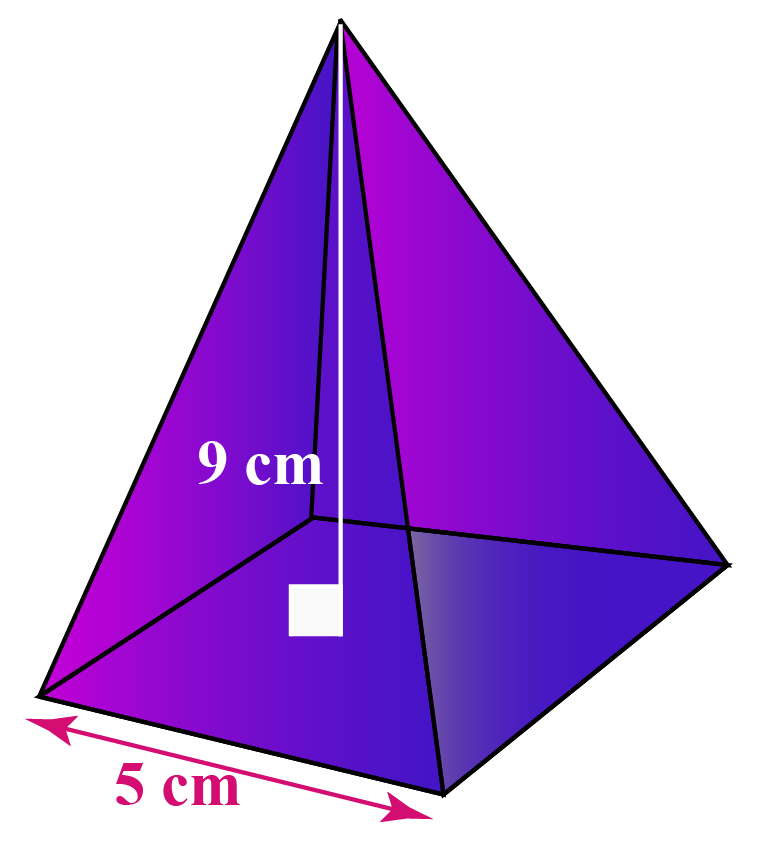Solution:

Pyramid volume  = \begin{align}\frac{1}{3} \times a^2 \times h \end{align} where $$a$$ is the length of the edge of the square.

Given,

• a = 5 cm
• h = 9 cm

Substituting the values, we get:

\begin{align} \text{Volume} &= \frac{1}{3} \times 5^2 \times 9 \\ &= \frac{1}{3} \times 25 \times 9 \\ &=75\: \text{cm}^3 \end{align}

 $$\therefore$$ Volume of the pyramid = 75 cm3
 Example 2

Cheops Pyramid in Egypt has a base measuring about 755 ft. $$\times$$ 755 ft. and the height is around 480 ft.

Calculate its volume.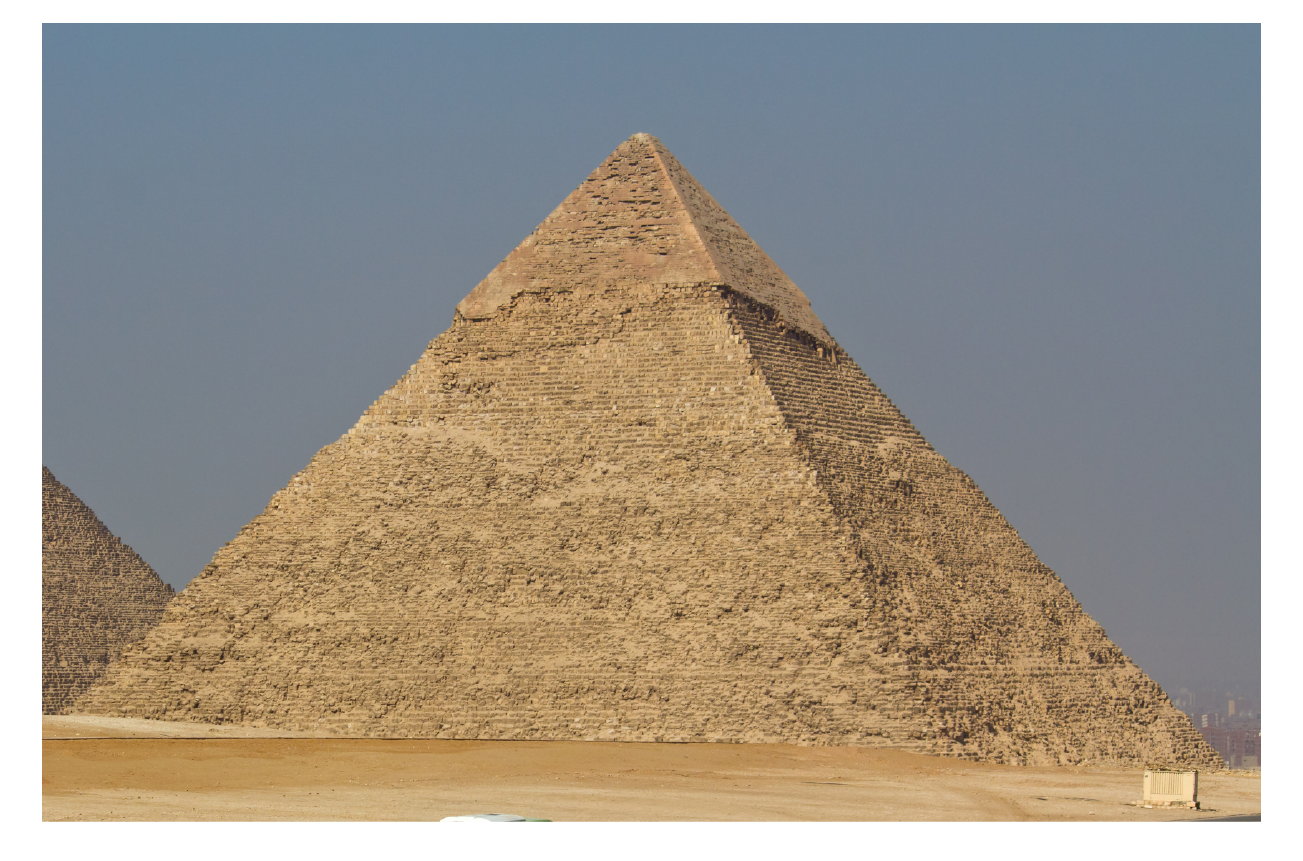Solution:

Since the base measures 755 ft. $$\times$$ 755 ft., the Cheops Pyramid is a square Pyramid.

Volume of a square pyramid = \begin{align} \frac{1}{3} \times a^2 \times h \end{align}

Substituting the values, we get:

\begin{align} \text{ Pyramid Volume} &=\frac{1}{3} \times 755 \times 755 \times 480 \\ &= \frac{1}{3} \times 570025 \times 480 \\ &=91204000 \:\text{cubic feet} \end{align}

 $$\therefore$$ Volume of Cheops Pyramid = 9,12,04,000 cubic ft.
 Example 3

A pyramid has a hexagon as its base of edge length 6 cm and height 9 cm.

Find its volume.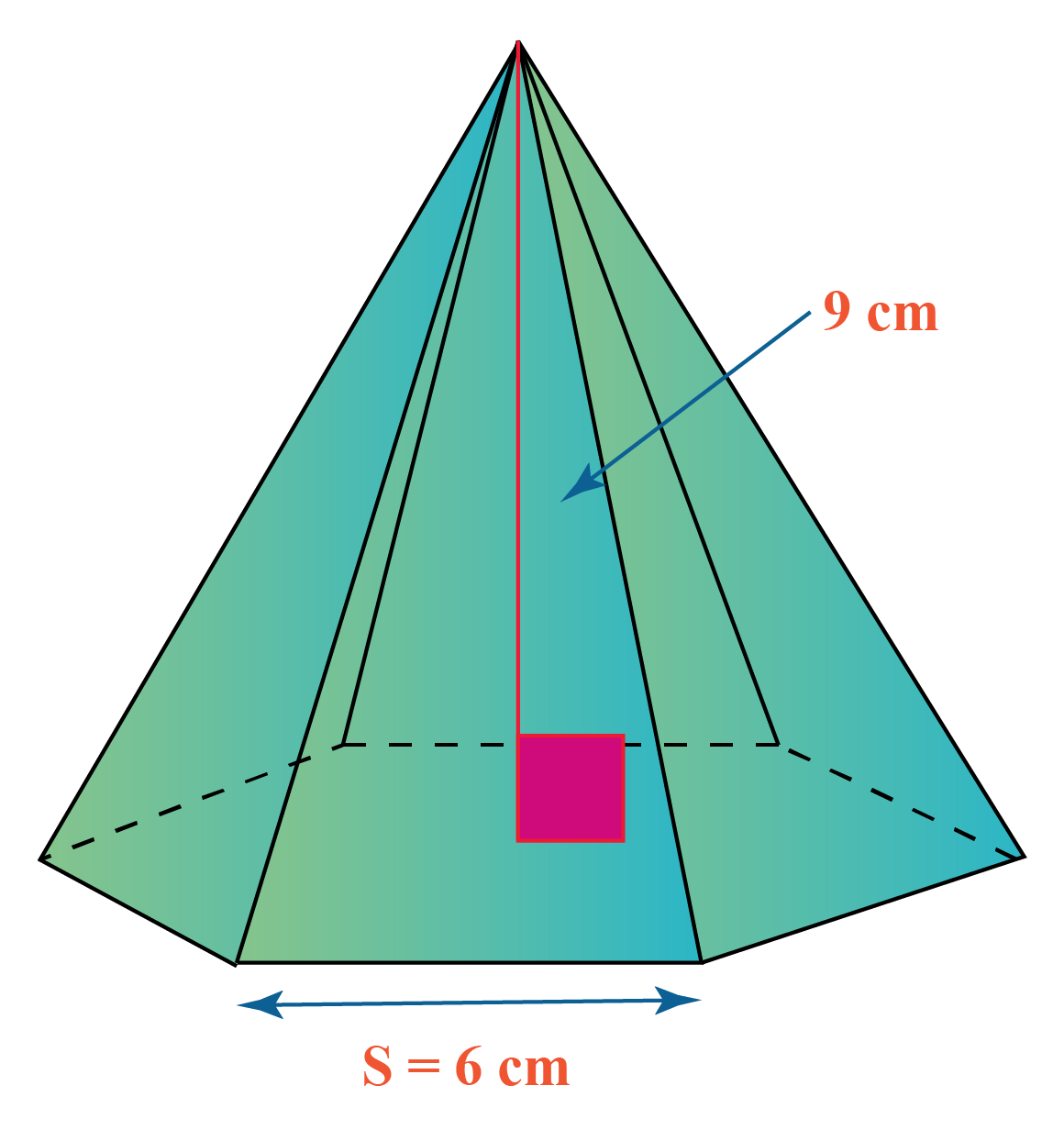Solution:

Volume of a hexagonal pyramid

\begin{align}\frac{\sqrt{3}}{2} \times a^2 \times h \end{align}

Given,

• a = 6
• h = 9

Substituting the values, we get:

\begin{align} &= \frac{\sqrt{3}}{2} \times 6 \times 6 \times 9 \\ &= \frac{\sqrt{3}}{2} \times1944 \\ &\approx 280.59\: \text{cm}^3 \end{align}

 $$\therefore$$Volume of hexagonal pyramid = 280.59 cm3
 Example 4

Which has a greater volume: A square pyramid of base 12 cm and height 10 cm or A pentagonal pyramid of base area 144 cm2 and height 10 cm?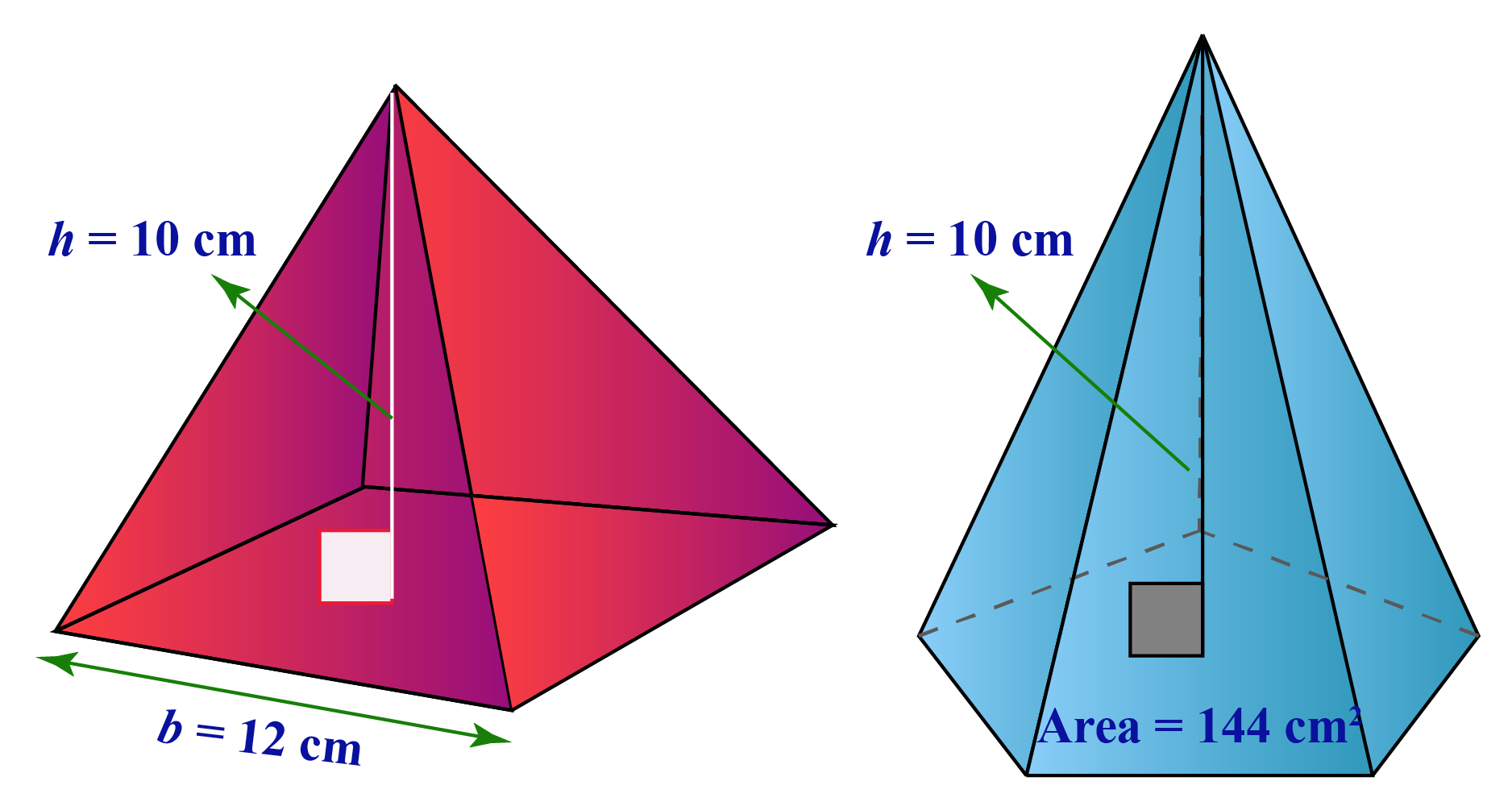Solution:

Volume of the Square Pyramid

\begin{align} \frac{1}{3} \times a^2 \times h \end{align}

where $$a$$ is the length of the edge of the square.

Given,

• a = 12 cm
• h = 10 cm

Substituting the value, we get:

Volume of the square pyramid:

\begin{align} &= \frac{1}{3} \times 12^2 \times 10 \\ &= \frac{1}{3} \times 144 \times 10 \\ &=480 \: \text{cm}^3 \end{align}

Volume of a Pentagonal Pyramid:

\begin{align} \frac{1}{3} \times \text{Base Area} \times \text{Height}\end{align}

Given,

• base area = 144 cm2
• height = 10 cm

Substituting the values,

Volume of a pentagonal pyramid:

\begin{align} &=\frac{1}{3} \times 144 \times 10 \\ &=480 \: \text{cm}^3 \end{align}

 $$\therefore$$ Volume of both pyramids are equal
 Example 5

Tim built a rectangular tent for his night camp.

The base of the tent is a rectangle of side 6 m $$\times$$ 10 m and the height is 3 m. What is the volume?Solution:

Volume of a rectangular pyramid is

\begin{align}\frac{1}{3} \times l \times b \times h \end{align}

where $$l$$ and $$b$$ are the length and breadth of the rectangle.

Given,

• $$l$$ = 6 m
• $$h$$ = 3 m
• $$b$$ = 10 m

Substituting the values, we get, pyramid volume:

\begin{align} &=\frac{1}{3} \times 6 \times 10 \times 3 \\ &= \frac{1}{3} \times 180 \\ &=60 \: \text{m}^3 \end{align}

 $$\therefore$$ Volume of the tent is 60 m3

You can use the volume of a pyramid worksheet at the end of this page to solve more problems.

Have a doubt that you want to clear? Get it clarified with simple solutions on Volume of a Pyramid from our Math Experts at Cuemath’s LIVE, Personalised and Interactive Online Classes.

Make your kid a Math Expert, Book a FREE trial class today!

## Practice Questions

Here are a few activities for you to practice. Select/Type your answer and click the "Check Answer" button to see the result.

IMO (International Maths Olympiad) is a competitive exam in Mathematics conducted annually for school students. It encourages children to develop their math solving skills from a competition perspective.

## 1. How do you find the volume of a pyramid?

Volume of a pyramid is one third the base area multiplied to the height of the pyramid.

Vpyramid = \begin{align}\frac{1}{3} \times \text{Base area} \times \text{Height}\end{align}

## 2. Is the volume of a pyramid a cube?

Volume is measured in cubic units.

For example, the volume of a square pyramid of base area 90 cmand height 10 cm is 300 cubic centimeter or 300 cm3

## 3. How do you calculate the volume of a triangular pyramid?

Volume of a triangular pyramid is one third the base area multiplied to the pyramid's height.

Vpyramid =  \begin{align}\frac{1}{3} \times \text{Base area} \times \text{Height}\end{align}

More Important Topics
Numbers
Algebra
Geometry
Measurement
Money
Data
Trigonometry
Calculus